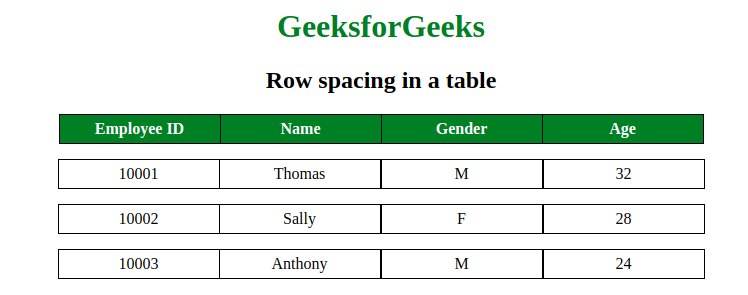# Space between two rows in a table using CSS?

• Last Updated : 30 Jul, 2021

The space between two rows in a table can be done using CSS border-spacing and border-collapse property. The border-spacing property is used to set the spaces between cells of a table and border-collapse property is used to specify whether the border of table is collapse or not. The border-spacing attribute can only be used if border-collapse attribute is set to separate.

Example:

Attention reader! Don’t stop learning now. Get hold of all the important HTML concepts with the Web Design for Beginners | HTML  course.

 ```<``html``>``    ``<``head``>``        ``<``style``>``            ``table {``                ``border-collapse: collapse;``            ``}``            ``th {``                ``background-color:green;``                ``Color:white;``            ``}``            ``th, td {``                ``width:150px;``                ``text-align:center;``                ``border:1px solid black;``                ``padding:5px``             ` `            ``}``            ``.geeks {``                ``border-right:hidden;``            ``}``            ``.gfg {``                ``border-collapse:separate;``                ``border-spacing:0 15px;``            ``}``            ``h1 {``                ``color:green;``            ``}``        ````    ````    ``<``body``>``        ``<``center``>``        ``<``h1``>GeeksforGeeks``        ``<``h2``>Row spacing in a table``        ``<``table``>``            ``<``tr``>``                ``<``th``>Employee ID``                ``<``th``>Name``                ``<``th``>Gender``                ``<``th``>Age``            ````        ````        ``<``table` `class` `= ``"gfg"``>``            ``<``tr``>``                ``<``td` `class` `= ``"geeks"``>10001``                ``<``td``>Thomas``                ``<``td``>M``                ``<``td``>32``            ````            ``<``tr``>``                ``<``td` `class` `= ``"geeks"``>10002``                ``<``td``>Sally``                ``<``td``>F``                ``<``td``>28``            ````            ``<``tr``>``                ``<``td` `class` `= ``"geeks"``>10003``                ``<``td``>Anthony``                ``<``td``>M``                ``<``td``>24``            ````        ````        ````    `````

Output:HTML is the foundation of webpages, is used for webpage development by structuring websites and web apps.You can learn HTML from the ground up by following this HTML Tutorial and HTML Examples.

CSS is the foundation of webpages, is used for webpage development by styling websites and web apps.You can learn CSS from the ground up by following this CSS Tutorial and CSS Examples.

My Personal Notes arrow_drop_up